Next: Gale-Berlekamp Switching Game Up: Hadamard Matrices Previous: Introduction

# Discrepancy and Ramsey Theory for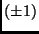-Matrices

Lemma 2.1   (Lindsey's Lemma) Let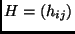be a Hadamard matrix. Let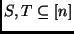and,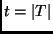. ThenDefinition 2.2   We call the submatrix on the entries corresponding to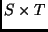anrectangle in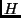. We call the sum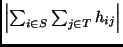the discrepancy of this rectangle.

Discrepancy measures the deviation from uniform distribution.Definition 2.3   A rectangle is homogeneous if all of its entries are equal.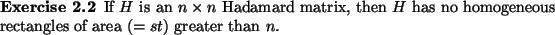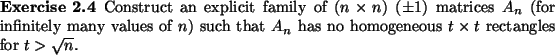OPEN PROBLEM 2.4   Construct an explicit family ofmatrices(for infinitely many values of) such thathas no homogeneousrectangles for.

Laszlo Babai 2003-06-19